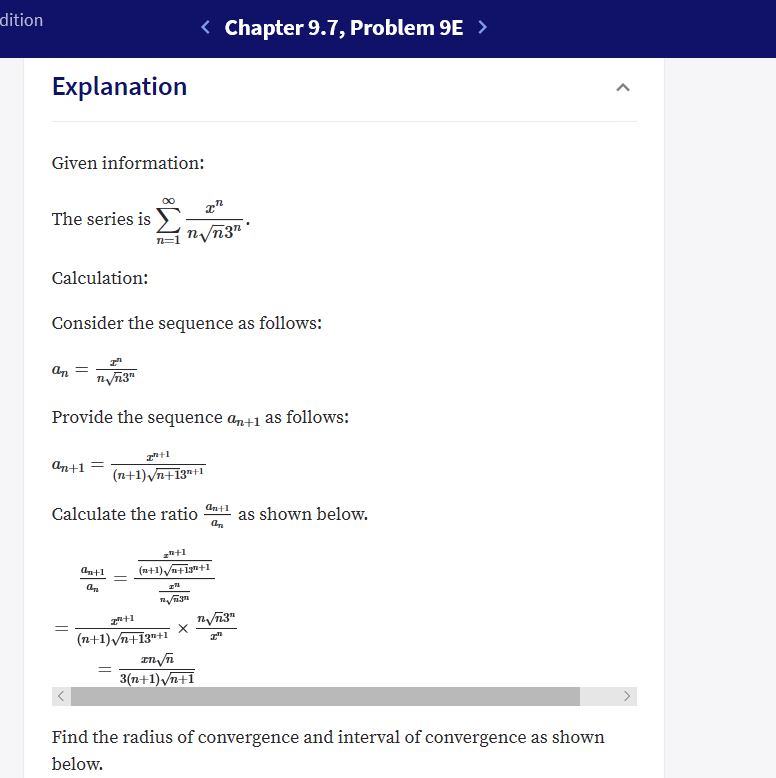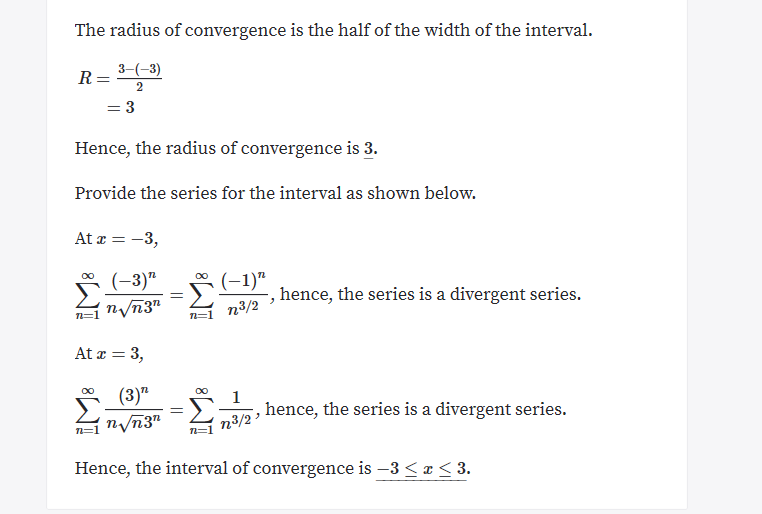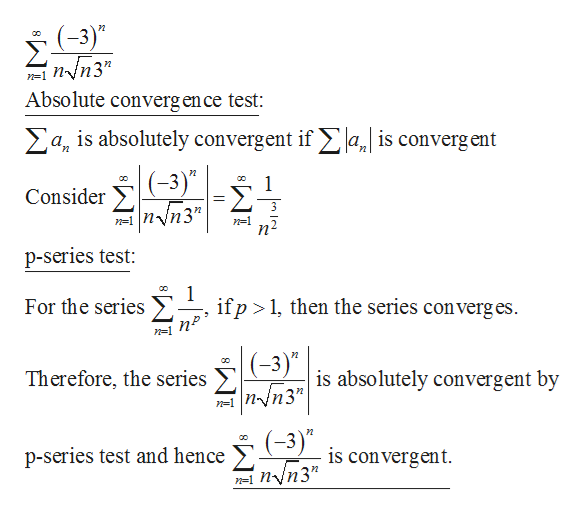# ditionChapter 9.7, Problem 9E >ExplanationGiven information:OOа"The series isnVn3"Calculation:Consider the sequence as follows:ат —пупз"Provide the sequence an+1 as follows:ап+1(n+1)Vn+13"1Calculate the ratioas shown below.(n+1)+1+1antlzа,3(n+1)Vn+13"+13(n+1) Vn+1Find the radius of convergence and interval of convergence as shownbelow. The radius of convergence is the half of the width of the interval3-3)2=3Hence, the radius of convergence is 3.Provide the series for the interval as shown below.At 3Σ(-3)"n/n3"OO(1)"-, hence, the series is a divergent series.n3/2n=1= 3At(3)"Σ.OOOO1hence, the series is a divergent series.nvn3"n3/2Hence, the interval of convergence is -3 < x < 3.

Question
1 views

Textbook : University Calculus

Section 9.7, problem #9

In this problem we have to find the series interval of convergence. Which i found to be -3<x<3, then i went to check the endpoints to see if they are included and when plugging in -3 or 3 for x i found those series to be divergent like how it says on this website, but then the answer on the website and in the back of the book says that the endpoints are included. Why are the endpoints included if both series come out to be divergent when testing the endpoints?help_outlineImage Transcriptionclosedition Chapter 9.7, Problem 9E > Explanation Given information: OO а" The series is nVn3" Calculation: Consider the sequence as follows: ат — пупз" Provide the sequence an+1 as follows: ап+1 (n+1)Vn+13"1 Calculate the ratio as shown below. (n+1)+1+1 antl z а, 3 (n+1)Vn+13"+1 3(n+1) Vn+1 Find the radius of convergence and interval of convergence as shown below. fullscreenhelp_outlineImage TranscriptioncloseThe radius of convergence is the half of the width of the interval 3-3) 2 =3 Hence, the radius of convergence is 3. Provide the series for the interval as shown below. At 3 Σ (-3)" n/n3" OO (1)" -, hence, the series is a divergent series. n3/2 n=1 = 3 At (3)" Σ. OO OO 1 hence, the series is a divergent series. nvn3" n3/2 Hence, the interval of convergence is -3 < x < 3. fullscreen
check_circle

Step 1

The given series is,

Step 2

At x = –3,help_outlineImage Transcriptionclose(-3)" пуп3" n-1 Absolute converg en ce test: a is absolutely convergent if , is convergent (-3 1 Consider =1nVn3" p-series test: Σ. 1 if p 1, then the series converges For the series (-3)" Σ n-1 nn3" is absolutely convergent by Therefore, the series (-3)" p-series test and hence is con vergent. fullscreen
Step 3

At x = 3,

...

### Want to see the full answer?

See Solution

#### Want to see this answer and more?

Solutions are written by subject experts who are available 24/7. Questions are typically answered within 1 hour.*

See Solution
*Response times may vary by subject and question.
Tagged in

### Other